# Parametric Binomial Example - Demonstrate MTTF

This example appears in the Life Data Analysis Reference book.

In this example, we will use the parametric binomial method to design a test that will demonstrate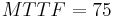$MTTF=75\,\!$ hours with a 95% confidence if no failure occur during the test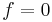$f=0\,\!$. We will assume a Weibull distribution with a shape parameter$\beta =1.5\,\!$. We want to determine the number of units to test for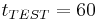${{t}_{TEST}}=60\,\!$ hours to demonstrate this goal.

The first step in this case involves determining the value of the scale parameter$\eta \,\!$ from the$MTTF\,\!$ equation. The equation for the$MTTF\,\!$ for the Weibull distribution is: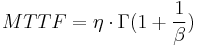$MTTF=\eta \cdot \Gamma (1+\frac{1}{\beta })\,\!$

where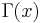$\Gamma (x)\,\!$ is the gamma function of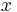$x\,\!$. This can be rearranged in terms of$\eta\,\!$: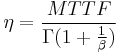$\eta =\frac{MTTF}{\Gamma (1+\tfrac{1}{\beta })}\,\!$

Since$MTTF\,\!$ and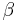$\beta \,\!$ have been specified, it is a relatively simple matter to calculate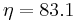$\eta =83.1\,\!$. From this point on, the procedure is the same as the reliability demonstration example. Next, the value of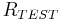${{R}_{TEST}}\,\!$ is calculated as: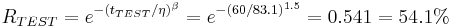${{R}_{TEST}}={{e}^{-{{({{t}_{TEST}}/\eta )}^{\beta }}}}={{e}^{-{{(60/83.1)}^{1.5}}}}=0.541=54.1%\,\!$

The last step is to substitute the appropriate values into the cumulative binomial equation. The values of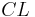$CL\,\!$,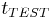${{t}_{TEST}}\,\!$,$\beta \,\!$,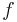$f\,\!$ and$\eta \,\!$ have already been calculated or specified, so it merely remains to solve the binomial equation for$n\,\!$. The value is calculated as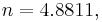$n=4.8811,\,\!$ or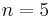$n=5\,\!$ units, since the fractional value must be rounded up to the next integer value. This example solved in Weibull++ is shown next.

The procedure for determining the required test time proceeds in the same manner, determining$\eta \,\!$ from the$MTTF\,\!$ equation, and following the previously described methodology to determine${{t}_{TEST}}\,\!$ from the binomial equation with Weibull distribution.